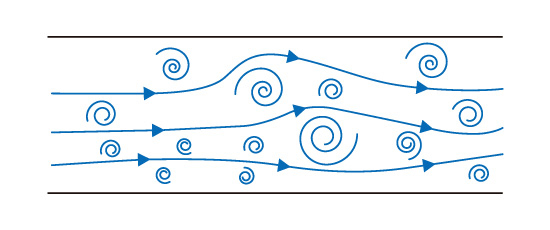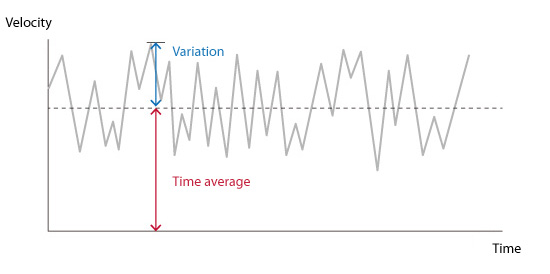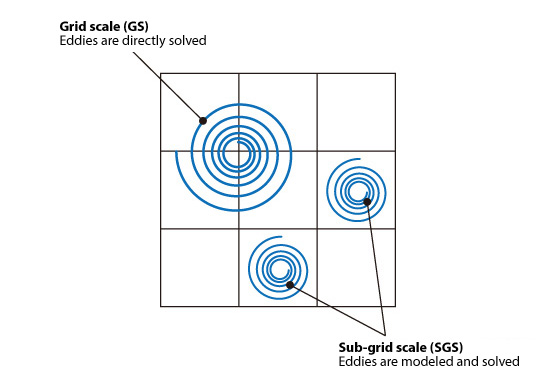# Basic Course of Thermo-Fluid Analysis 18: Chapter 5 Basics of thermo-fluid analyses - 5.9.1 Reynolds-averaged Navier–Stokes (RANS), 5.9.2 Large eddy simulation (LES)

#### 5.9 Turbulent flow analysis

As previously described , most flows in real life are turbulent flows . Figure 5.23 shows a simplified representation of a turbulent flow. Swirling pockets of higher energy flows called eddies are generated when the flow is turbulent. Eddies repeatedly split into smaller eddies. As a result, eddies of many sizes are generated in a flow field and will affect the flow and temperature distributions. The eddies continue to split and eventually dissipate into thermal energy due to viscosity and disappear.Figure 5.23: Image of turbulent flows

Three different methods are generally used for modeling turbulence depending on how eddies are treated with different scales.

• Reynolds-averaged Navier–Stokes (RANS)
• Large eddy simulation (LES)
• Direct numerical simulation (DNS)

#### 5.9.1 Reynolds-averaged Navier–Stokes (RANS)

For many engineering flow analyses, averaged flow, temperature distribution, and forces acting on an object are often sufficient to make engineering decisions. Therefore, as shown in Figure 5.24, an averaging method is used to time average the turbulent phenomenon, which is intrinsically transient. This averaging operation is called Reynolds averaging.Figure 5.24 Reynolds averaging

Reynolds-averaged Navier-Stokes (RANS) is a method used to obtain the average flow by using the Reynolds-averaged equations.
The Reynolds-averaged governing equations contain a term called the Reynolds stress, which represents the effect of turbulence on an average flow. However, the Reynolds stress cannot be obtained by only using averaged equations. An additional approximation is necessary to express Reynolds stress. A turbulence model is generally used for the approximation. The standard k-EPS model is a well-known turbulence model.
RANS cannot simulate the change of a flow field over time or the structure of turbulent eddies (e.g., a transient flow that releases eddies, such as a Karman vortex), which cannot be calculated using an averaged flow. One primary advantage of RANS is that the calculation load is the lowest among the aforementioned three methods. Thus, RANS is widely used in practical calculations.

#### 5.9.2 Large eddy simulation (LES)

Larger eddies have more energy than smaller eddies and will have more effect on a flow field. A second method for modeling turbulence is to filter the fundamental equations based on eddy size. Eddies greater than the filtering threshold are directly solved and do not have to be modeled. Eddies smaller than the threshold are modeled and their effect is calculated. In summary, eddies are filtered based on their size. Only eddies remaining after the filtration are directly solved. This method is called large eddy simulation (LES).
In general, a mesh width is regarded as an appropriate filter size. The larger scale used for direct calculation is called grid scale (GS), while the smaller scale to be modeled is called sub-grid scale (SGS).Figure 5.25: Grid scales and sub-grid scales

When the mesh size is small, more eddies are directly solved and fewer eddies need to be modeled. In this case, calculation load increases. However, the obtained result is likely more accurate.